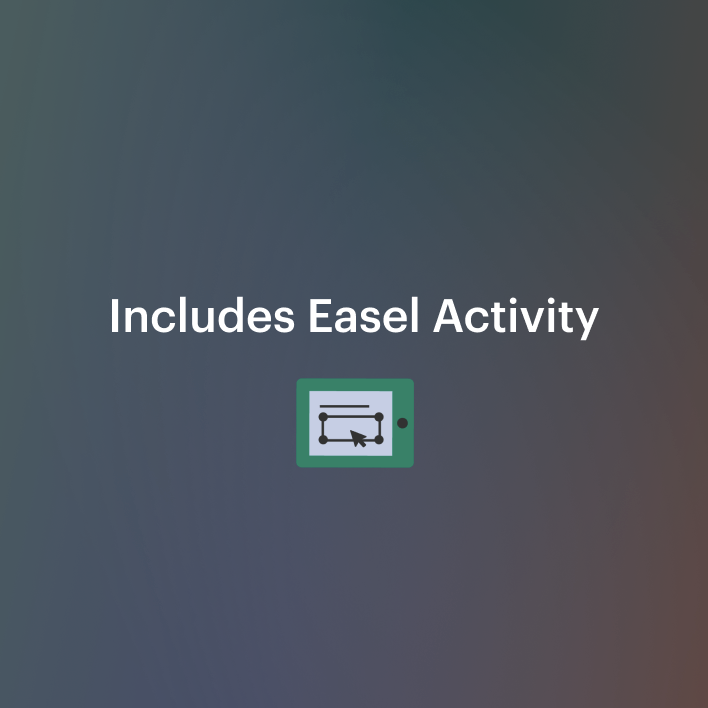# Geometry Unit: Lines, Angles, and Triangles

Rated 4.85 out of 5, based on 65 reviews
65 Ratings3rd - 5th, Homeschool
Subjects
Resource Type
Standards
Formats Included
• PDF
Pages
42 pages
Report this resource to TPT
Easel Activity Included
This resource includes a ready-to-use interactive activity students can complete on any device.  Easel by TPT is free to use! Learn more.

### Description

If you are looking for geometry lessons for third, fourth, or fifth grade, this unit will be useful for you. This geometry unit contains math worksheets and multi-modal activities for the following concepts:

✅ intersecting lines
✅ parallel lines
✅ perpendicular lines
✅ lines
✅ line segments
✅ rays
✅ acute angles
✅ obtuse angles
✅ right angles
✅ isosceles right triangles
✅ equilateral acute triangles
✅ scalene obtuse angles
✅ congruent figures

This unit includes a total of 5 visual and kinesthetic activities for students, and 4 worksheets. These lessons should take about a week to complete.

This geometry unit contains:

✅ Types of line relationships demonstration and activity
✅ Types of lines demonstration and activity
✅ Types of angles demonstration and activities
✅ Classifying triangles homework sheet (2 pages, with answer key)
✅ Triangles exploration (with answer key)
✅ Types of angles worksheet
✅ Lines worksheet
✅ Line pairs in figures worksheet (with answer key)
✅ Types of triangles worksheet (with answer key)
✅ Geometry open response question worksheet

Update: Teachers Pay Teachers has included a digital overlay. This means you can upload it directly to your Google Classroom from this site. Students can type answers in the boxes provided. They can use the pen tool to circle chosen answers.

The materials that you will need to complete the activities with your class are:
► Clear tape
► Popsicle sticks
► Permanent markers (orange, red, blue)
► Colored construction paper

✨✨✨ Are you looking for more ways to help your students excel in and enjoy geometry? I have a whole range of geometry items here! Check out these popular products. ✨✨✨

Geometry Mini Unit: 3 Dimensional Figures
Snowflake Project for Geometry
Coordinate-points-and-ordered-pairs-robot-project
Measuring Degrees of Angles Task Cards and Activities
Parallel and Perpendicular Line Pairs Robots
Drawing Perpendicular and Parallel Lines with Tools
Drawing Parallel and Perpendicular Lines with Tools Project

Total Pages
42 pages
Included
Teaching Duration
1 Week
Report this resource to TPT
Reported resources will be reviewed by our team. Report this resource to let us know if this resource violates TPT’s content guidelines.

### Standards

to see state-specific standards (only available in the US).
Draw points, lines, line segments, rays, angles (right, acute, obtuse), and perpendicular and parallel lines. Identify these in two-dimensional figures.
Classify two-dimensional figures based on the presence or absence of parallel or perpendicular lines, or the presence or absence of angles of a specified size. Recognize right triangles as a category, and identify right triangles.
Recognize angles as geometric shapes that are formed wherever two rays share a common endpoint, and understand concepts of angle measurement:
An angle is measured with reference to a circle with its center at the common endpoint of the rays, by considering the fraction of the circular arc between the points where the two rays intersect the circle. An angle that turns through 1/360 of a circle is called a “one-degree angle,” and can be used to measure angles.
An angle that turns through 𝘯 one-degree angles is said to have an angle measure of 𝘯 degrees.